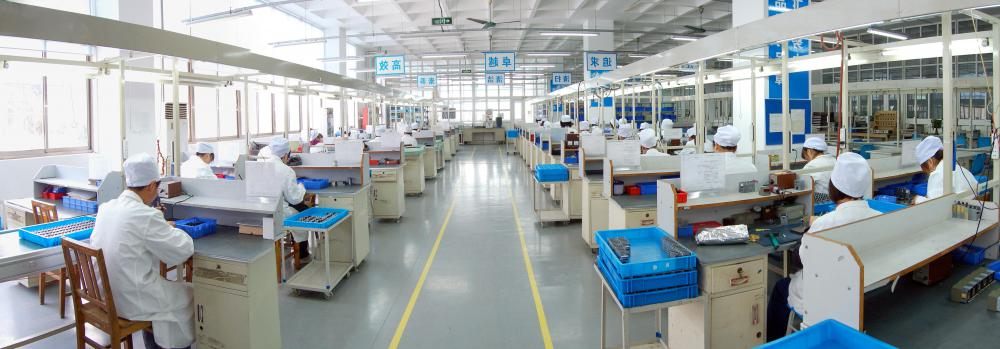# What is a Contribution Margin?

John Lister
John Lister

The contribution margin measures the amount of overall profit that is added by each sale. This is the difference the sale makes; it is also possible that the change it makes is to reduce losses rather than increase profits. The figure could also be negative, meaning each sale reduces overall profits, though this would be a sign of a serious problem with its business set-up.

The figure can be expressed in three different ways. The total contribution margin is the total revenue minus the variable costs. Variable costs are those which are associated specifically with production and thus could include the cost of raw materials; they don't include fixed costs such as factory hire. The total contribution margin therefore shows how much is left over from sales to help offset, and hopefully exceed, fixed costs.In most cases, the variable cost per unit produced is lower when more items are manufactured.

The unit contribution margin measures the unit revenue minus the variable cost per unit. In other words, it's the amount the company receives for each unit sold, minus the specific costs associated with producing that unit. The amount the company receives won't necessarily be the price the end user pays, as the product may pass through a retailer. The unit contribution margin can thus show how profitable a particular product line is. It can also show how the level by which sales would have to increase to have a specific effect on overall company profits.

The contribution margin ratio is the unit contribution margin divided by the unit price. By definition, the same figure can be worked out by dividing the total contribution margin by total revenue. The figure effectively shows what proportion of the sales price is left over after variable costs to go towards covering fixed costs and eventually making a profit.

The most common way to display the concept is in a graph displaying the way increased sales affect overall profitability. Every sale adds some profits to the company. Of course, at first these sales are not enough to cover the fixed costs and the company will be in a loss-making position. As the number of sales goes up, eventually the profits from the sales covers the fixed costs and the company will break even. After this point, increased sales add overall profits.

There are some limitations to using the concept in calculations. Most significantly, it works on the assumption that the production costs per unit are consistent. In practice the more units a firm produces, the lower its variable costs per unit. This is because of economies of scale, such as getting a discount on raw materials when bought in bulk.

## You might also Like